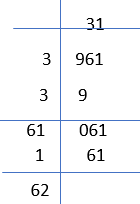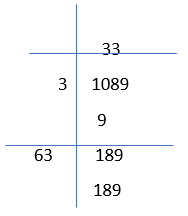# Worksheet on Square Root using Long Division Method | Square Root by Long Division Method Worksheets

Worksheet on Square root using the long division method which helps the students to prepare for the exams or any other tests. To determine the long division method, we will first divide the digits of the number into pairs of segments, starting with the digit in the units place. Then identify each pair and the remaining final number. And next, we will divide the digits into segments, which will be starting from the left side of the segment.

The number which is largest and whose square is equal to or less than the first segment is called as divisor which is also called as quotient. Then we will subtract the square of the divisor from the first segment and bring down the next segment to the right of the reminder to get the dividend. And in this step, the new divisor is obtained by taking two times the previous quotient. In the final step, we will repeat the above steps until all the segments have been taken and the quotient so obtained will be the required square root of the given number. Here, below we can see the examples on square root using the long division method with solutions.

1. Evaluate using the long division method: √961

Solution:

We will perform the long division method for the number 961 and the square root of the 961 is
961= 31×312. Evaluate using the long division method: √1089

Solution:

The square root of the number 1089 is
1089= 33×33.
Here in the below, we can see the long division method3. Evaluate using the long division method: √10404

Solution:

The square root of the number 10404 is
10404= 102×1024. What will be the least number which must be subtracted from 3,606 to make it a perfect square

Solution:

As the square of 60 is 3600, and by subtracting 3606-3600= 6. And 6 is the least number that must be subtracted.

5. What will be the least number that must be subtracted from 8,125 to obtain a perfect square.

Solution:

As the square of 90 is 8,100, and by subtracting 8,125-8,100= 25. And 25 is the least number that must be subtracted.

6. What will be the least number which must be added to 61,500 to make it a perfect square.

Solution:

To find the least number, we will add 61,500 to obtain a perfect square.
So √61500= 247.99,
And the square root is 247.99. So, the perfect square is 248.
So 248×248= 61,504.
And the least number which must be added is 61,504-61,500= 4.
So the least number which should be added is 4.

7. What will be the least number that must be added to 6,200 to obtain a perfect square.

Solution:

To find the least number, we will add 6,200 to obtain a perfect square.
we know that
78×78= 6,084
79×79= 6,241
6,241 is in between the squares of the numbers 78 and 79.
Consider,
6,241- 6,084= 157
And the least number that must be added is 157.